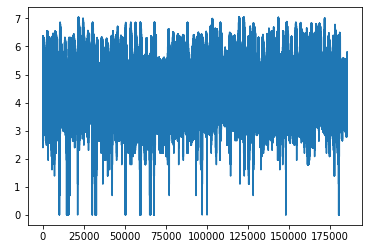# .fit loss nan

I have the problem that find_lr() only runs partially or not at all.
-and fit() loss is nan.
Someone somewhere in the forum mentioned exploding gradient so I checked my data and it looked alright.
Continous variables have mean ~0 and std ~1.
Using log, the y_range is between 0-7.But the plot was wrong, there was no warning about infinite values.

I saw that np.mean(yl) = -inf. So some y values are zero, which means np.log(0) = -inf
Hard-learned lesson: Sanity check in the future, if yl.min() = -inf then throw error.

Is this an appropriate solution? If log of y is less than zero then set to zero.

``````yl = np.log(y)
yl[yl < 0.0] = 0.0``````

Have you tried np.log1p ?